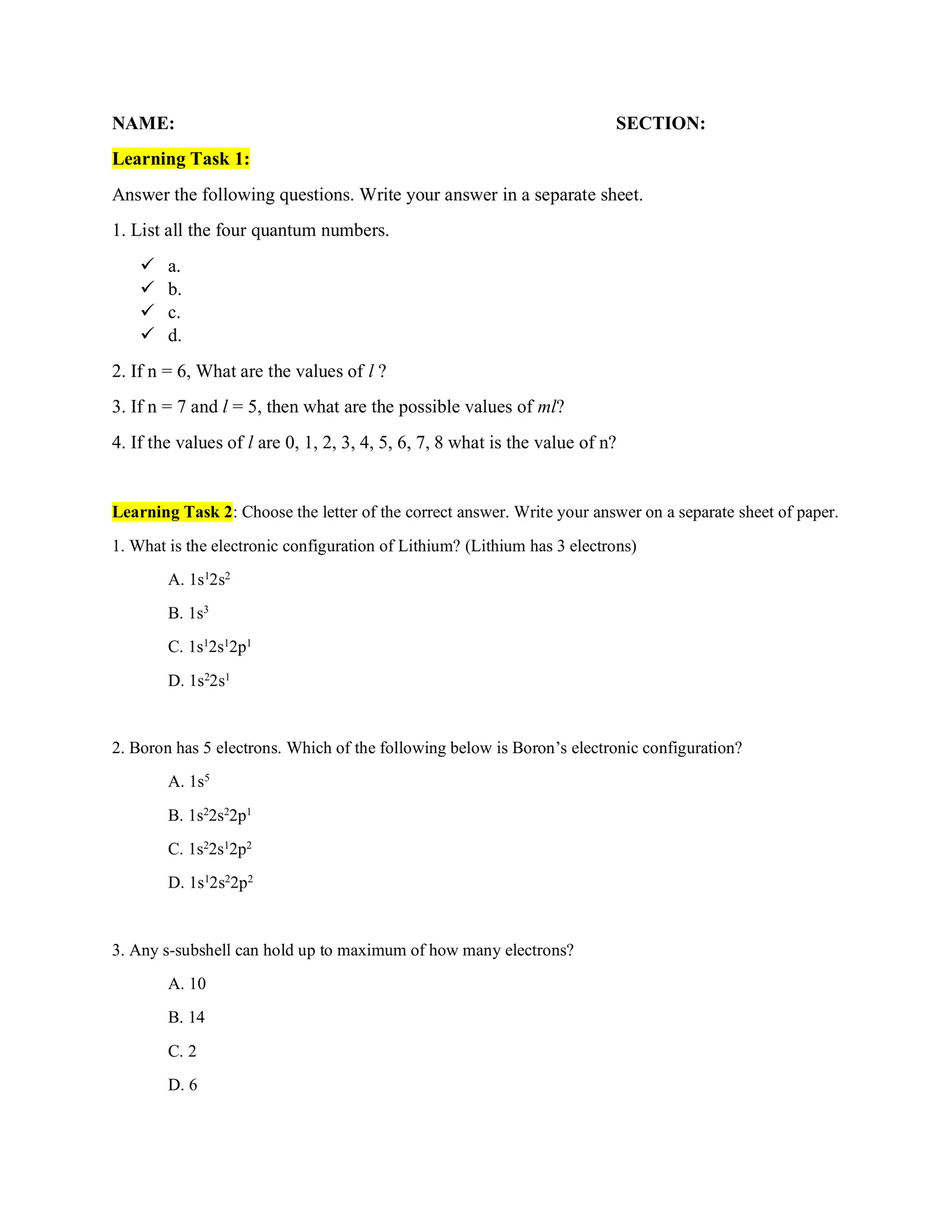# Quantum and Electron Configuration 9 Ponce

Created
##### Pages

NAME: SECTION: Learning Task 1: Answer the following questions. Write your answer in a separate sheet. 1. List all the four quantum numbers. ✓ a. ✓ b. ✓ c. ✓ d. 2. If n = 6, What are the values of l ? 3. If n = 7 and l = 5, then what are the possible values of ml? 4. If the values of l are 0, 1, 2, 3, 4, 5, 6, 7, 8 what is the value of n? Learning Task 2: Choose the letter of the correct answer. Write your answer on a separate sheet of paper. 1. What is the electronic configuration of Lithium? (Lithium has 3 electrons) A. 1s12s2 B. 1s3 C. 1s12s12p1 D. 1s22s1 2. Boron has 5 electrons. Which of the following below is Boron’s electronic configuration? A. 1s5 B. 1s22s22p1 C. 1s22s12p2 D. 1s12s22p2 3. Any s-subshell can hold up to maximum of how many electrons? A. 10 B. 14 C. 2 D. 6